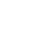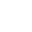> > Equation of Parabola

# Equation of Parabola

The set of all points in a plane that are equidistant from a fixed line and a fixed point (not on the fixed line) in the plane is a parabola. The fixed line is the ‘directrix’ and the fixed point is the ‘focus’ {Fig. 1(a)}. In simple words, it is the shape described when we throw a ball in the air.

Further, a line through the focus and perpendicular to the directrix is the axis of the parabola. Also, the point of intersection of the parabola with the axis is the vertex {Fig. 1(b)}.

###Suggested VideosIntroduction to Conic SectionsTerms Associated to ConicsDiameter of a Parabola## Standard Equations of the Parabola

When the vertex of a parabola is at the ‘origin’ and the axis of symmetry is along the x or y-axis, then the equation of the parabola is the simplest. Here is a quick look at four such possible orientations:Of these, let’s derive the equation for the parabola shown in Fig.2 (a). As can be seen in the diagram, the parabola has focus at (a, 0) with a > 0. Also, the directrix x = – a. Let F be the focus and l, the directrix. Also, let FM be perpendicular to the directrix and bisect FM at point O. Produce MO to X.### Browse more Topics Under Conic Sections

By the definition of the parabola, the mid-point O is on the parabola and is called the vertex of the parabola. Next, take O as origin, OX the x-axis and OY perpendicular to it as the y-axis. Let the distance from the directrix to the focus be 2a. Then, the coordinates of the focus are: (a, 0), and the equation of the directrix is x + a = 0, as shown in the diagram below:## Derivation of the Equation

Now, we take a point P(x, y) on the parabola such that, PF = PB … (1) … where PB is perpendicular to l. The coordinates of the point B are (- a, y). Also, by the distance formula, we know that

PF = √ {(x – a)2 + y2}
Also, PB = √ (x + a)2

Since, PF = PB [from eq. (1)], we get
√ {(x – a)2 + y2} = √ (x + a)2

So, by squaring both sides, we have (x – a)2 + y2 = (x + a) or, x2 – 2ax + a2 + y2 = x2 + 2ax + a. Further, by the solving the equation, we have y2 = 4ax … where a > 0. Therefore, we can say that any point on the parabola satisfies the equation:

y2 = 4ax … (2)

Let’s look at the converse situation now. If P(x, y) satisfies equation (2), then

PF = √ {(x – a)2 + y2}
= √ {(x – a)2 + 4ax} … using the RHS of equation (2)
= √ (x2 – 2ax + a2 + 4ax) = √ (x2 + 2ax + a2)
= √ (x + a)= PB … (3)

Hence, we conclude that the point P(x, y) satisfying eq. (2) lies on the parabola. Also, equations (2) and (3) prove that the equation to the parabola with vertex at the origin, focus at (a, 0) and directrix x = – a, is y2 = 4ax.

#### Important

Since a > 0, x can assume any positive value or zero but no negative value. Hence, the curve extends indefinitely far into the first and the fourth quadrants. The axis of the parabola is the positive x-axis.

Similarly, we can derive the equations of the parabolas in the other three figures (Fig. 2) as:

• (b): y2 = – 4ax,
• (c): x2 = 4ay,
• (d): x2 = – 4ay.

These four equations are the Standard Equations of Parabolas.

### Observations

• A parabola is symmetric with its axis. If the equation has a y2 term, then the axis of symmetry is along the x-axis and if the equation has an x2 term, then the axis of symmetry is along the y-axis.
• For axis of symmetry along the x-axis:
• It opens to the right if the coefficient of x is positive
• It opens to the left if the coefficient of x is negative
• For axis of symmetry along the y-axis:
• It opens upwards if the coefficient of y is positive
• It opens downwards if the coefficient of y is negative

## Latus Rectum

Latus rectum of a parabola is a line segment perpendicular to the axis of the parabola, through the focus and whose endpoints lie on the parabola as shown below.Let’s find the length of the latus rectum of the parabola y2 = 4ax. Refer the diagram given below.By definition of the parabola; AF = AC. However, it can be seen in the diagram above that AC = FM (since both AC and FM are perpendicular to the directrix and AF is perpendicular to the x-axis). Also, FM = 2a. Hence, we can conclude that AF = 2a. Further, the figure is symmetric with respect to the x-axis. Hence, the length of the latus rectum,

AB = AF + FB = 2a + 2a = 4a.

## Solved Examples for You

Question: Find the coordinates of the focus, axis, the equation of the directrix and latus rectum of the parabola y2 = 8x.

Solution: To begin with, the equation is given in y2. Hence, the axis of symmetry is along the x-axis. Secondly, the coefficient of x is positive. Hence, it opens to the right (refer the sub-topic observations in this article above). And finally, by comparing y2 = 8x with y2 = 4ax, we get a = 2. Which means that the focus of the parabola is 2.

Further, the equation of the directrix is x = – a. Hence, by replacing hte value of a, we get x = – 2. Also, the length of the latus rectum is 4a = 4 x 2 = 8. This is how the diagram looks like:Share with friends

## Customize your course in 30 seconds

##### Which class are you in?
5th
6th
7th
8th
9th
10th
11th
12th
Get ready for all-new Live Classes!
Now learn Live with India's best teachers. Join courses with the best schedule and enjoy fun and interactive classes.Ashhar Firdausi
IIT Roorkee
BiologyDr. Nazma Shaik
VTU
ChemistryGaurav Tiwari
APJAKTU
Physics
Get Started

## Browse

##### Conic Sections2 Followers

Most reacted comment
1 Comment authorsRecent comment authors
Subscribe
Notify ofGuest
Dami

Nigeria not among

## Question Mark?

Have a doubt at 3 am? Our experts are available 24x7. Connect with a tutor instantly and get your concepts cleared in less than 3 steps.